BGG Top 50 Statistics : from 01 Nov 06 to 01 Dec 06
For each game currently in the BGG Top 50, an overview is given for the difference in following 5 categories :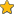RankingRatingVotesOwnersPlays

The difference is calculated based on the 2 dates mentioned in the GeekList title.
The highest and lowest differences per category are also mentioned.

Please give me your feedback (/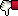) on this list as I'm thinking about making this a monthly feature.
• Posted Sat Dec 2, 2006 10:07 am
• [+] Dice rolls
1 , 2  Next »    |
1. Board Game: Puerto Rico [Average Rating:8.01 Overall Rank:24]Ranking : from 1 to 1 = 0

Rating : from 8.46 to 8.45 = -0.01

Votes : from 6944 to 7158 = +214

Owners : from 7041 to 7256 = +215

Plays : from 13859 to 14664 = +805
• [+] Dice rolls
{{error.message}}
{{comment.error.message}}
Pg. {{commentParams.pageid}} » {{data.config.endpage}}
2. Board Game: Tigris & Euphrates [Average Rating:7.70 Overall Rank:80]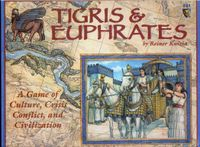Ranking : from 2 to 2 = 0

Rating : from 8.22 to 8.21 = -0.01

Votes : from 4325 to 4449 = +124

Owners : from 4469 to 4592 = +123

Plays : from 6740 to 7015 = +275
• [+] Dice rolls
{{error.message}}
{{comment.error.message}}
Pg. {{commentParams.pageid}} » {{data.config.endpage}}
3. Board Game: Caylus [Average Rating:7.79 Overall Rank:55]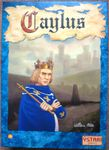Ranking : from 3 to 3 = 0

Rating : from 8.2 to 8.18 = -0.02 (= highest rating decrease)

Votes : from 2775 to 2927 = +152

Owners : from 2711 to 2878 = +167

Plays : from 6561 to 6964 = +403
• [+] Dice rolls
{{error.message}}
{{comment.error.message}}
Pg. {{commentParams.pageid}} » {{data.config.endpage}}
4. Board Game: Power Grid [Average Rating:7.87 Overall Rank:36]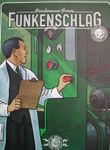Ranking : from 4 to 4 = 0

Rating : from 8.16 to 8.16 = 0

Votes : from 3426 to 3578 = +152

Owners : from 3347 to 3491 = +144

Plays : from 7205 to 7663 = +458
• [+] Dice rolls
{{error.message}}
{{comment.error.message}}
Pg. {{commentParams.pageid}} » {{data.config.endpage}}
5. Board Game: El Grande [Average Rating:7.76 Overall Rank:61]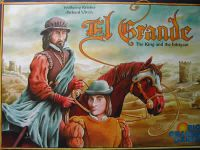Ranking : from 5 to 5 = 0

Rating : from 8.06 to 8.06 = 0

Votes : from 3219 to 3338 = +119

Owners : from 3354 to 3484 = +130

Plays : from 2824 to 3022 = +198
• [+] Dice rolls
{{error.message}}
{{comment.error.message}}
Pg. {{commentParams.pageid}} » {{data.config.endpage}}
6. Board Game: The Princes of Florence [Average Rating:7.54 Overall Rank:157]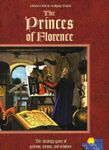Ranking : from 6 to 6 = 0

Rating : from 8.03 to 8.02 = -0.01

Votes : from 3076 to 3162 = +86

Owners : from 3120 to 3203 = +83

Plays : from 3645 to 3818 = +173
• [+] Dice rolls
{{error.message}}
{{comment.error.message}}
Pg. {{commentParams.pageid}} » {{data.config.endpage}}
7. Board Game: Die Macher [Average Rating:7.62 Overall Rank:254]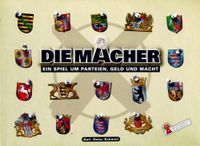Ranking : from 7 to 7 = 0

Rating : from 7.92 to 7.96 = +0.04 (= highest rating increase)

Votes : from 799 to 850 = +51

Owners : from 1018 to 1166 = +148

Plays : from 569 to 646 = +77
• [+] Dice rolls
{{error.message}}
{{comment.error.message}}
Pg. {{commentParams.pageid}} » {{data.config.endpage}}
8. Board Game: Age of Steam [Average Rating:7.75 Overall Rank:118]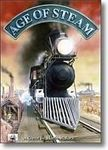Ranking : from 9 to 8 = +1

Rating : from 7.91 to 7.92 = +0.01

Votes : from 1707 to 1750 = +43

Owners : from 1668 to 1706 = +38

Plays : from 2479 to 2594 = +115
• [+] Dice rolls
{{error.message}}
{{comment.error.message}}
Pg. {{commentParams.pageid}} » {{data.config.endpage}}
9. Board Game: Ra [Average Rating:7.47 Overall Rank:162]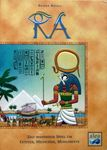Ranking : from 8 to 9 = -1

Rating : from 7.92 to 7.92 = 0

Votes : from 2912 to 3004 = +92

Owners : from 2788 to 2910 = +122

Plays : from 7444 to 7897 = +453
• [+] Dice rolls
{{error.message}}
{{comment.error.message}}
Pg. {{commentParams.pageid}} » {{data.config.endpage}}
10. Board Game: Commands & Colors: Ancients [Average Rating:7.78 Overall Rank:120]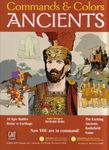Ranking : from 10 to 10 = 0

Rating : from 7.86 to 7.87 = +0.01

Votes : from 743 to 809 = +66

Owners : from 1008 to 1079 = +71

Plays : from 1956 to 2216 = +260
• [+] Dice rolls
{{error.message}}
{{comment.error.message}}
Pg. {{commentParams.pageid}} » {{data.config.endpage}}
11. Board Game: War of the Ring [Average Rating:7.77 Overall Rank:106]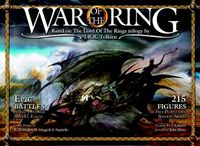Ranking : from 11 to 11 = 0

Rating : from 7.85 to 7.86 = +0.01

Votes : from 2075 to 2156 = +81

Owners : from 2614 to 2711 = +97

Plays : from 2264 to 2338 = +74
• [+] Dice rolls
{{error.message}}
{{comment.error.message}}
Pg. {{commentParams.pageid}} » {{data.config.endpage}}
12. Board Game: Twilight Struggle [Average Rating:8.31 Overall Rank:8] [Average Rating:8.31 Unranked]Ranking : from 12 to 12 = 0

Rating : from 7.83 to 7.84 = +0.01

Votes : from 600 to 642 = +42

Owners : from 703 to 767 = +64

Plays : from 1372 to 1459 = +87
• [+] Dice rolls
{{error.message}}
{{comment.error.message}}
Pg. {{commentParams.pageid}} » {{data.config.endpage}}
13. Board Game: Goa [Average Rating:7.59 Overall Rank:152]Ranking : from 13 to 13 = 0

Rating : from 7.83 to 7.83 = 0

Votes : from 1970 to 2054 = +84

Owners : from 1933 to 2022 = +89

Plays : from 3536 to 3671 = +135
• [+] Dice rolls
{{error.message}}
{{comment.error.message}}
Pg. {{commentParams.pageid}} » {{data.config.endpage}}
14. Board Game: Wallenstein [Average Rating:7.48 Overall Rank:478]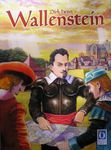Ranking : from 14 to 14 = 0

Rating : from 7.81 to 7.81 = 0

Votes : from 1466 to 1500 = +34

Owners : from 1391 to 1413 = +22

Plays : from 2537 to 2614 = +77
• [+] Dice rolls
{{error.message}}
{{comment.error.message}}
Pg. {{commentParams.pageid}} » {{data.config.endpage}}
15. Board Game: Paths of Glory [Average Rating:8.05 Overall Rank:135]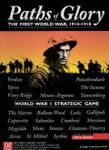Ranking : from 17 to 15 = +2

Rating : from 7.75 to 7.77 = +0.02

Votes : from 667 to 683 = +16

Owners : from 1085 to 1108 = +23

Plays : from 416 to 435 = +19
• [+] Dice rolls
{{error.message}}
{{comment.error.message}}
Pg. {{commentParams.pageid}} » {{data.config.endpage}}
16. Board Game: Hannibal: Rome vs. Carthage [Average Rating:7.81 Overall Rank:203]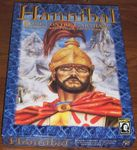Ranking : from 15 to 16 = -1

Rating : from 7.78 to 7.76 = -0.02 (= highest rating decrease)

Votes : from 609 to 625 = +16

Owners : from 736 to 748 = +12 (= lowest owners increase)

Plays : from 406 to 417 = +11
• [+] Dice rolls
{{error.message}}
{{comment.error.message}}
Pg. {{commentParams.pageid}} » {{data.config.endpage}}
17. Board Game: Memoir '44 [Average Rating:7.56 Overall Rank:128] [Average Rating:7.56 Unranked]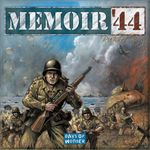Ranking : from 16 to 17 = -1

Rating : from 7.76 to 7.75 = -0.01

Votes : from 3166 to 3289 = +123

Owners : from 3359 to 3474 = +115

Plays : from 9995 to 10647 = +652
• [+] Dice rolls
{{error.message}}
{{comment.error.message}}
Pg. {{commentParams.pageid}} » {{data.config.endpage}}
18. Board Game: Railways of the World [Average Rating:7.69 Overall Rank:115] [Average Rating:7.69 Unranked]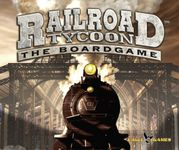Ranking : from 18 to 18 = 0

Rating : from 7.73 to 7.74 = +0.01

Votes : from 1272 to 1354 = +82

Owners : from 1298 to 1378 = +80

Plays : from 2532 to 2694 = +162
• [+] Dice rolls
{{error.message}}
{{comment.error.message}}
Pg. {{commentParams.pageid}} » {{data.config.endpage}}
19. Board Game: YINSH [Average Rating:7.69 Overall Rank:172] [Average Rating:7.69 Unranked]Ranking : from 20 to 19 = +1

Rating : from 7.72 to 7.73 = +0.01

Votes : from 966 to 1001 = +35

Owners : from 1059 to 1091 = +32

Plays : from 2570 to 2717 = +147
• [+] Dice rolls
{{error.message}}
{{comment.error.message}}
Pg. {{commentParams.pageid}} » {{data.config.endpage}}
20. Board Game: Go [Average Rating:7.64 Overall Rank:154]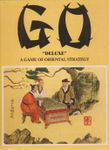Ranking : from 19 to 20 = -1

Rating : from 7.73 to 7.72 = -0.01

Votes : from 1753 to 1804 = +51

Owners : from 2081 to 2142 = +61

Plays : from 4531 to 4794 = +263
• [+] Dice rolls
{{error.message}}
{{comment.error.message}}
Pg. {{commentParams.pageid}} » {{data.config.endpage}}
21. Board Game: Catan [Average Rating:7.17 Overall Rank:365] [Average Rating:7.17 Unranked]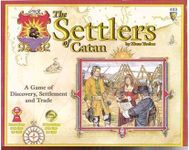Ranking : from 21 to 21 = 0

Rating : from 7.68 to 7.67 = -0.01

Votes : from 8027 to 8261 = +234 (= highest votes increase)

Owners : from 8326 to 8591 = +265

Plays : from 12197 to 12974 = +777
• [+] Dice rolls
{{error.message}}
{{comment.error.message}}
Pg. {{commentParams.pageid}} » {{data.config.endpage}}
22. Board Game: Modern Art [Average Rating:7.38 Overall Rank:220]Ranking : from 22 to 22 = 0

Rating : from 7.68 to 7.67 = -0.01

Votes : from 2491 to 2545 = +54

Owners : from 2574 to 2631 = +57

Plays : from 3246 to 3349 = +103
• [+] Dice rolls
{{error.message}}
{{comment.error.message}}
Pg. {{commentParams.pageid}} » {{data.config.endpage}}
23. Board Game: Taj Mahal [Average Rating:7.30 Overall Rank:396]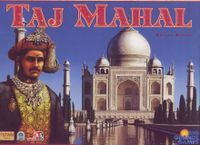Ranking : from 23 to 23 = 0

Rating : from 7.65 to 7.67 = +0.02

Votes : from 1633 to 1692 = +59

Owners : from 1692 to 1801 = +109

Plays : from 1827 to 1944 = +117
• [+] Dice rolls
{{error.message}}
{{comment.error.message}}
Pg. {{commentParams.pageid}} » {{data.config.endpage}}
24. Board Game: Hammer of the Scots [Average Rating:7.55 Overall Rank:403]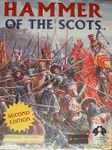Ranking : from 25 to 24 = +1

Rating : from 7.65 to 7.65 = 0

Votes : from 852 to 877 = +25

Owners : from 1079 to 1107 = +28

Plays : from 887 to 923 = +36
• [+] Dice rolls
{{error.message}}
{{comment.error.message}}
Pg. {{commentParams.pageid}} » {{data.config.endpage}}
25. Board Game: Samurai [Average Rating:7.44 Overall Rank:199]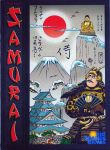Ranking : from 24 to 25 = -1

Rating : from 7.65 to 7.65 = 0

Votes : from 2686 to 2769 = +83

Owners : from 2692 to 2767 = +75

Plays : from 4890 to 5182 = +292
• [+] Dice rolls